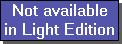The SDL Component Suite is an industry leading collection of components supporting scientific and engineering computing. Please visit the SDL Web site for more information....## How to use TPLSModelThe class TPLSModel implements the partial least squares (PLS) regression technique (PLS2 using SIMPLS, to be specific). This class supports both PLS regression and PLS discriminant analysis, the distinction between both methods is controled by the property IsDiscriminantModel.

In order to create and use the PLS regression model you have first to load the training data into the array properties XMat and YMat. By default, XMat and YMat are 1x1-matrices which have to be resized before loading the data. The matrix XMat contains the independent variables ("descriptors"), with the rows being the objects and the columns being the variables. The response variables have to be loaded into the matrix YMat. Finally, you have to assign the number of desired factors into the property Factors and you have to select the proper scaling mode by setting the property ScalingMode.

 Hint: Please note that the maximum number of (mathematically) allowed and meaningful factors can be obtained by the method MaxFactors. However, MaxFactors can return the correct maximum number of factors only after having adjusted the input matrix XMat to the proper size.

After setting up the model parameters and loading the data into XMat and YMat, the model can be calculated by calling the method CalculateModel. This will fill in the regression coefficients (property RegCoeff) as well a bunch of other matrices which contain additional information on the PLS model:

If the model has been computed successfully, you can use it to predict response values of unknown samples by calling the method Predict. A calculated model can be stored on disk by the method SaveModelCoefficients and loaded from disk by LoadModelCoefficients.

In order to cross validate the model the method CrossValidateModel has to be called. Please be prepared that the cross validation may take considerable time (depending on the size of the data matrices and the number of factors). Thus it is a good idea to use the event OnPercentDone to provide some feedback to the user. Further, the event OnCheckCVDAbort can be used to abort the cross validation process.

Following is a sample code torso showing the principal usage of the PLS model (it is assumed that the number of objects, and the number of independent variables, and the number of response variables are contained in the variables no_of_objects, no_of_input_vars, and no_of_response_vars, respectively):

```var
PLSMod : TPLSModel;

...
...
PLSMod := TPLSModel.Create(nil);       // create the PLS model instance
// resize the data matrices
PLSMod.XMat.Resize(no_of_input_vars, no_of_objects);
PLSMod.YMat.Resize(no_of_response_vars, no_of_objects);
for iy:=1 to no_of_objects do
begin
for ix:=1 to no_of_input_vars do
PLSMod.XMat[ix,iy] := ...          // copy the independent data
for ix:=1 to no_of_response_vars do
PLSMod.XMat[ix,iy] := ...          // copy the response data
end;
PLSMod.ScalingMode := scmCenter;       // scaling mode of independent variables
nfac := 20;                            // 20 factors should be sufficient for most cases
if PLSMod.MaxFactors < nfac then       // restrict number of factors if required
nfac := PLSMod.MaxFactors;

PLSMod.CalculateModel;                 // now calculate the model

YHat := TMatrix.Create(nil);           // apply the model to calculate the
PLSMod.Predict(PLSMod.XMat,YHat);      // estimated y values; in the same way
...                                 // real unknowns could be processed by
...                                 // the PLS model

PLSMod.Free;                           // finally free up the memory
...
...
```
Alternatively, the TPLSModel component could by taken from the component palette and put on a form. In this case the Create and Free method must not be called (they are called automatically when the form is created or destroyed).

Last Update: 2013-May-14